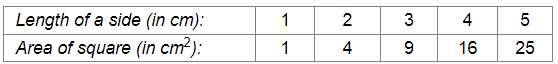# The following table gives the information regarding length of a side of a square and its area:

Question:

The following table gives the information regarding length of a side of a square and its area:Draw a graph to illustrate this information.

Solution:

Here, length of a side is an independent variable and area of square is a dependent variable. So, we take length of a side on the x-axis and area of square on the y-axis.

Let us choose the following scale:

On x-axis: 2 cm = 1 cm

On y-axis: 1 cm = 2 cm2

Now we plot (1,1), (2,4), (3,9), (4,16), (5,25). These points are joined to get the graph representing the given information as shown in the figure below.​